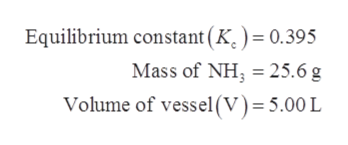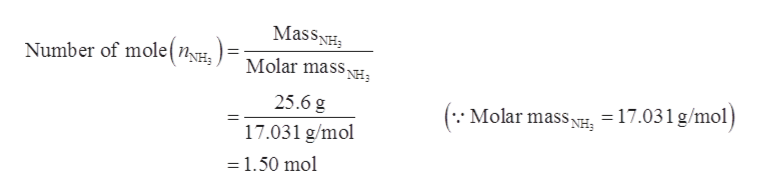# For the reaction 2 NH3(g) ⇋ N2(g) + 3H2(g), Kc= 0.395 at 350 degrees Celsius. A sample of NH3 of mass 25.6 g is laced in a reaction vessel of volume 5.00 L and heated to 350 degrees Celsius. What are the equilibrium concentrations of NH3, N2, and H2?

Question
66 views

For the reaction 2 NH3(g) ⇋ N2(g) + 3H2(g), Kc= 0.395 at 350 degrees Celsius. A sample of NH3 of mass 25.6 g is laced in a reaction vessel of volume 5.00 L and heated to 350 degrees Celsius. What are the equilibrium concentrations of NH3, N2and H2?

check_circle

Step 1

The equilibrium constant of a reaction is calculated by the help of equilibrium concentrations of the components and their stoichiometric coefficients.

Step 2

Given data:help_outlineImage TranscriptioncloseEquilibrium constant (K.) = 0.395 Mass of NH 25.6 g Volume of vessel(v)= 5.00 L fullscreen
Step 3

The number of moles of NH3 is ca...help_outlineImage TranscriptioncloseMassNH Number of mole(n Molar massNH; 25.6 g 17.031 g/mol) Molar mass 17.031 g/mol 1.50 mol fullscreen

### Want to see the full answer?

See Solution

#### Want to see this answer and more?

Solutions are written by subject experts who are available 24/7. Questions are typically answered within 1 hour.*

See Solution
*Response times may vary by subject and question.
Tagged in

### Physical Chemistry